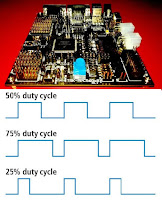# Freescale/NXP MPC5606B: eMIOS0 Testing Program (PWM)In this example,

- We will be testing eMIOS by generating 6 PWM output simultaneously and will be changing its duty cycle in run time using switch inputs.

Program Outcome:
```20KHz PWM at
eMIOS0_1 => PA,   eMIOS0_2 => PA,
eMIOS0_3 => PA,   eMIOS0_4 => PA,
eMIOS0_5 => PA,   eMIOS0_6 => PA.

Initial Duty_Cycle = 10%
Press Switch1   ->   Duty_Cycle = 20%
Press Switch2   ->   Duty_Cycle = 40%
Press Switch3   ->   Duty_Cycle = 60%
Press Switch4   ->   Duty_Cycle = 80%```

Changelog:
```26/03/2016: Bug:Phase lag of 1uSec is present between (eMIOS0_1,2,3) and (eMIOS0_4,5,6)
01/04/2016: Phase lag removed. [clear the GTBE bit at beginning of eMIOS_Init() and set it at the end of the eMIOS_Init().]```

Connections:
```SWITCH No. => Port No. => Pad No. => Pin No.(144-pin LQFP package)
SWITCH1    => PE    => PCR => PIN 10
SWITCH2    => PE    => PCR => PIN 12
SWITCH3    => PE    => PCR => PIN 128
SWITCH4    => PE    => PCR => PIN 129

eMIOS No.  => Port No. => Pad No. => Pin No.(144-pin LQFP package)
eMIOS0_1   => PA   =>  PCR  => PIN 11
eMIOS0_2   => PA   =>  PCR  => PIN 9
eMIOS0_3   => PA   =>  PCR  => PIN 90
eMIOS0_4   => PA   =>  PCR  => PIN 43
eMIOS0_5   => PA   =>  PCR  => PIN 118
eMIOS0_6   => PA   =>  PCR  => PIN 119```

Calculations:
```Frequency Calculation of PWM:
For "x" Hz Frequency, B_VAL = 1/[(x)*10^-6]
Duty Cycle Calculation of PWM:
For "y" % Duty Cycle, A_VAL = (y)*100/(x)```

Testing:- Output PWM waveform can be observed on DSO.

Exercise:
Make the connections shown below:
- Connect DSO probe +ve terminal to eMIOS0_1 PA and DSO prob gnd to the gnd of the TRK-MPC5606B board.
- Similarly, Connect remaining eMIOS0 and test it.

`eMIOS_Testing_TRK-MPC5606B_APDaga`